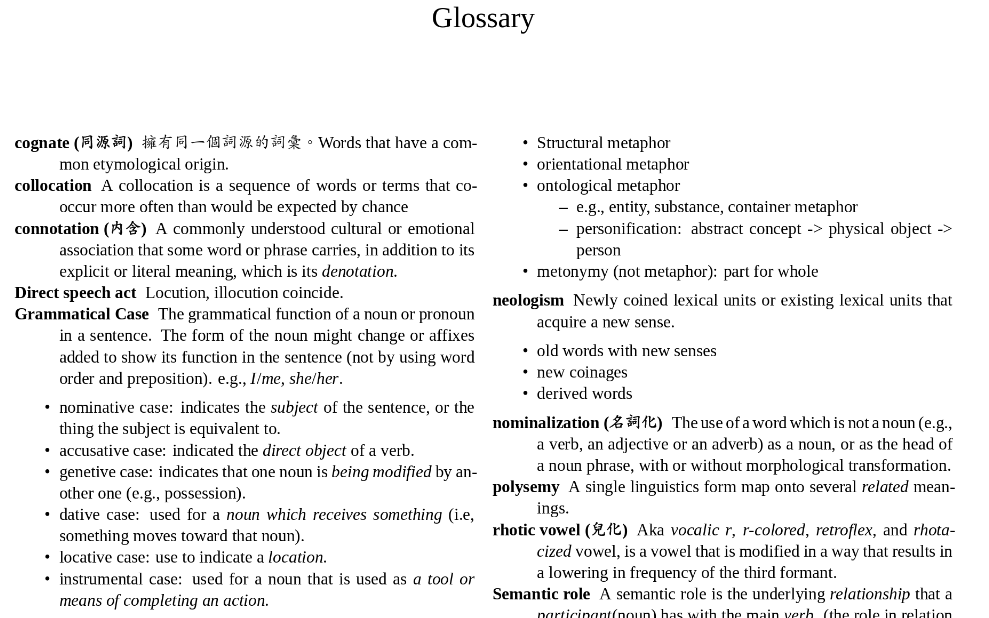Yongfu's Blog

# Create a Glossary in R Markdown

Oct 24, 2018

I was thinking about creating a glossary in bookdown and found out that there was already an issue about it. I like Yihui’s recommendation: use Pandoc’s definition lists. This was exactly what I had been doing, but I quickly found out that there was a major drawback – the definition lists won’t order alphabetically unless written in that way.

So I wrote an R function to reorder the definition lists written in R Markdown. Note that this functions only works for R Markdown files containing defintion lists exclusively. If the R Markdown files aren’t whole-definition-lists, the function will fail.

## Usage

To order the definition lists alphabetically, simply put the Rmd file path in the function. To have a different output file, provide the output file path as the second argument.

``````sort_def_list("glossary.Rmd")
# sort_def_list("glossary.Rmd", "reordered.Rmd")``````

The output in PDF looks like this (I used the `multicol` package)1:## Source Code

``````sort_def_list <- function(in_file, out_file = NULL) {
library(stringr)
library(dplyr)

# Extract, remove yaml header
yaml <- which(data == "---")
head <- c(data[yaml:yaml], "\n")
data <- data[(yaml+1):length(data)]

# Indexing lines
def_start <- which(stringr::str_detect(data,  "^: ")) - 1
def_end <- c(def_start[2:length(def_start)] - 1, length(data))

def_ranges <- dplyr::data_frame(term = data[def_start],
start = def_start,
end = def_end) %>%
dplyr::arrange(term) %>%
dplyr::mutate(new_start =
cumsum(
c(1, (end-start+1)[-length(term)])
)
) %>%
dplyr::mutate(new_end = new_start + (end-start))

# Create ordered definition list
data2 <- rep(NA, length(data))
for (i in seq_along(def_ranges\$term)) {
start <- def_ranges\$start[i]
end <- def_ranges\$end[i]
n_start <- def_ranges\$new_start[i]
n_end <- def_ranges\$new_end[i]
data2[n_start:n_end] <- data[start:end]
}

# Rewrite rmd
if (is.null(out_file)) out_file <- in_file
data2 <- c(head, data2[!is.na(data2)])
writeLines(paste(data2, collapse = "\n"),
out_file)
}``````
1. To see the source R Markdown file, visit `glossary.rmd`. To see the output PDF, visit `glossary.pdf`↩︎

Visit R-bloggers
Last updated: 2020-02-13